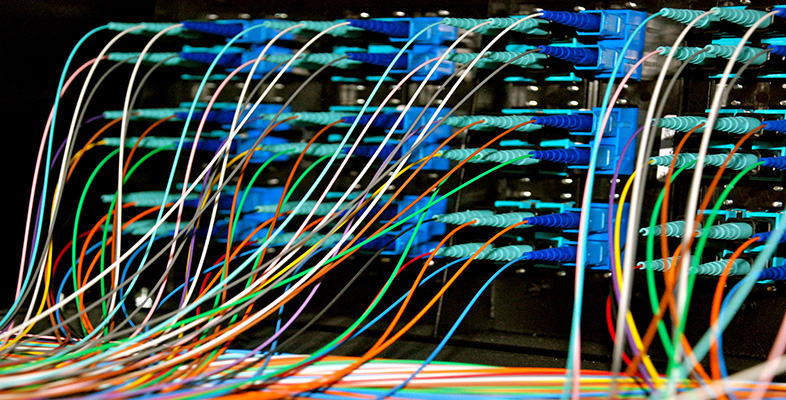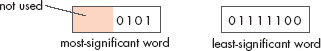Representing and manipulating data in computers

Start this free course now. Just create an account and sign in. Enrol and complete the course for a free statement of participation or digital badge if available.

Free course

# 2.2.4 Positive integers: encoding larger integers

The examples and activities in this section have looked only at 8-bit numbers. They have illustrated all of the principles of encoding positive integers as binary numbers without introducing the complication of larger numbers. But of course with 8 bits only relatively small integers can be encoded.

## Activity 8 (Self assessment)

What is the largest positive integer that can be encoded using just 8 bits?

The largest 8-bit binary number is 1111 1111. This is

(1 × 128) + (1 × 64) + (1 × 32) + (1 × 16) + (1 × 8) + (1 × 4) +  (1 × 2) + (1 × 1)

which is 255. So this is the largest positive integer than can be encoded.

There is a pattern to how many different integers can be encoded by a given number of bits. You have just seen in Activity 8 that with 8 bits (one byte) only the denary integers 0 to 255 can be encoded. This is 256 different integers in all. So 8 bits can encode 256 different integers, and 256 is 28. If you check, you will find that with 4 bits 24 (16) different integers can be encoded, with 6 bits, 26 (64) different integers. This leads to the general rule that with n bits 2n different integers can be encoded.

Using this general rule, 16 bits can encode 216 different positive integers, which is 65 536. So the denary integers 0 to 65 535 can be encoded in 16 bits, which is 2 bytes. Similarly 32 bits can encode 232 different positive integers, which is 4 294 967 296. So the denary integers 0 to 4 294 967 295 can be represented in 32 bits, which is 4 bytes.

## Activity 9 (Exploratory)

Did you notice that I said that with 2 bytes 65 536 different positive integers can be encoded, and then that they are 0 to 65 535? Why did I not say that they are 0 to 65 536?

### Discussion

In this method of encoding, a code of all zeros always represents denary 0. This leaves only 65 536 − 1 = 65 535 other patterns of 0s and 1s to represent other integers. So only the 65 535 integers 1 to 65 535 can be represented along with the 0.

The kitchen scales have been designed so that when they are weighing in metric they can weigh up to 3000 grams, in whole numbers of grams. I'm going to ignore for the moment the fact that the kitchen scales can handle both positive and negative integers, which is an added complication that I shall come to in Section 2.4. Imagine that the scales did not have the add-and-weigh facility and so did not need to deal with negative numbers. Then they would simply need to be able to encode positive integers from 0 to 3000.

## Activity 10 (Exploratory)

How many bits are needed to encode positive integers from 0 to 3000? Hint: you already know that 8 bits would offer too few codes (28 = 256) and 16 too many (2 = 65 536). So try some numbers between 8 and 16.

### Discussion

Eleven bits would offer 2048 different codes, which is too few. Twelve bits offer 4096, which is more than enough. So 12 bits are needed to encode positive integers from 0 to 3000.

Now it so happens that the computer in the kitchen scales uses 8-bit words. So how could it cope with the fact that 12 bits are needed to hold the codes for the weights in grams? The answer is very simple: two words would be used. One word would hold the rightmost 8 bits of the code, the other word the leftmost 4 (with some spare bits that are not used.) Figure 2 illustrates this for the code 0101 0111 1100. Note that the word on the left in Figure 2 is the most significant, as it holds the most-significant bits of the code word. The one on the right is the least significant.Figure 2 The 12-bit code word 0101 0111 1100 is held in two 8-bit data words

Provided the program had been carefully designed to take account of this arrangement, everything would work just fine.

A representation that uses more than one word is known as a multiple-length representation.

## Activity 11 (Self assessment)

What is the denary equivalent of the binary word

0101 0110

if it represents

1. a natural binary number

2. a binary-coded decimal number with two code words packed into the single 8-bit word?

1. The 8-bit number is equivalent to

(0 × 128) + (1 × 64) + (0 × 32) + (1 × 16) + (0 × 8) + (1 × 4) +  (1 × 2) + (0 × 1)

which is 86 in denary.

2. The 8-bit number is now equivalent to denary 5 then denary 6, which is denary 56.

## Box 2: Binary-coded decimal

The coding system described in Section 2.2 is known as the natural binary coding system. It uses the binary counting numbers 0, 1, 10, 11, 100, etc., to encode the denary counting numbers 0, 1, 2, 3, 4, etc.

There is an alternative coding system which is sometimes used. It is known as binary-coded decimal or BCD. Here each digit of a denary number is coded by its 4-bit binary equivalent. This results in as many 4-bit code words as there are digits in the original denary number. So for example denary 25 is encoded as:

0010 (two) then 0101 (five)

and denary 139 as:

0001 (one) then 0011 (three) then 1001 (nine).

In a computer with, say, a 16-bit word length, four BCD code words can be packed into a single computer word if desired. Alternatively each code word can form the least-significant four bits of a 16-bit word, with the other bits set to 0. It is important to make a decision on this point in each individual application, and then adhere to it.